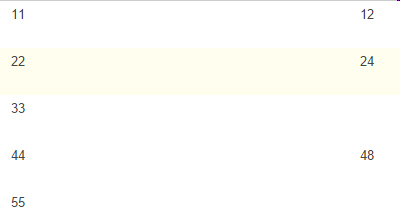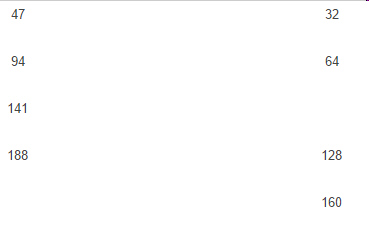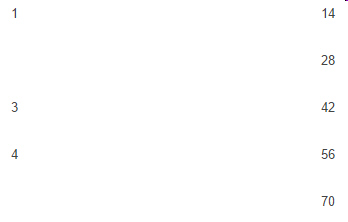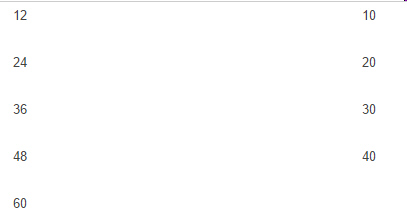6.RP.3.A Test 1B
 Name:    6.RP.3.A Test 1B

Multiple Response
Identify one or more choices that best complete the statement or answer the question.

1.Choose the 2 answers that complete the ratio table.
 a. 60 c. 72 b. 36 d. 30

2.a. 128 c. 512 b. 520 d. 120

3.Choose the two numbers that complete the ratio table.
 a. 235 c. 96 b. 72 d. 237

4.Choose the 2 correct answers that will complete the ratio table.
 a. 12 c. 6 b. 20 d. 24

5.Choose the 2 answers that complete the ratio table.
 a. 5 c. 10 b. 2 d. 7

Completion
Complete each statement.

6.What number completes the chart?

7.What number completes the chart?

8.What number completes the chart?

9.What number completes the chart?